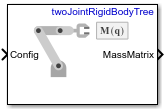# Joint Space Mass Matrix

Joint-space mass matrix for robot configuration

•Libraries:
Robotics System Toolbox / Manipulator Algorithms

## Description

The Joint Space Mass Matrix block returns the joint-space mass matrix for the given robot configuration (joint positions) for the Rigid body tree robot model.

## Ports

### Input

expand all

Robot configuration, specified as a vector of positions for all nonfixed joints in the robot model, as set by the Rigid body tree parameter. You can also generate this vector for a complex robot using the `homeConfiguration` or `randomConfiguration` functions inside a Constant or MATLAB Function block.

### Output

expand all

Joint-space mass matrix for the given robot configuration, returned as a positive-definite symmetric matrix.

## Parameters

expand all

Robot model, specified as a `rigidBodyTree` object. You can also import a robot model from an URDF (Unified Robot Description Formation) file using `importrobot`.

The default robot model, `twoJointRigidBodyTree`, is a robot with revolute joints and two degrees of freedom.

• `Interpreted execution` — Simulate model using the MATLAB® interpreter. This option shortens startup time but has a slower simulation speed than ```Code generation```. In this mode, you can debug the source code of the block.

• `Code generation` — Simulate model using generated C code. The first time you run a simulation, Simulink® generates C code for the block. The C code is reused for subsequent simulations, as long as the model does not change. This option requires additional startup time, but the speed of the subsequent simulations is comparable to ```Interpreted execution```.

Tunable: No

## Version History

Introduced in R2018a